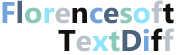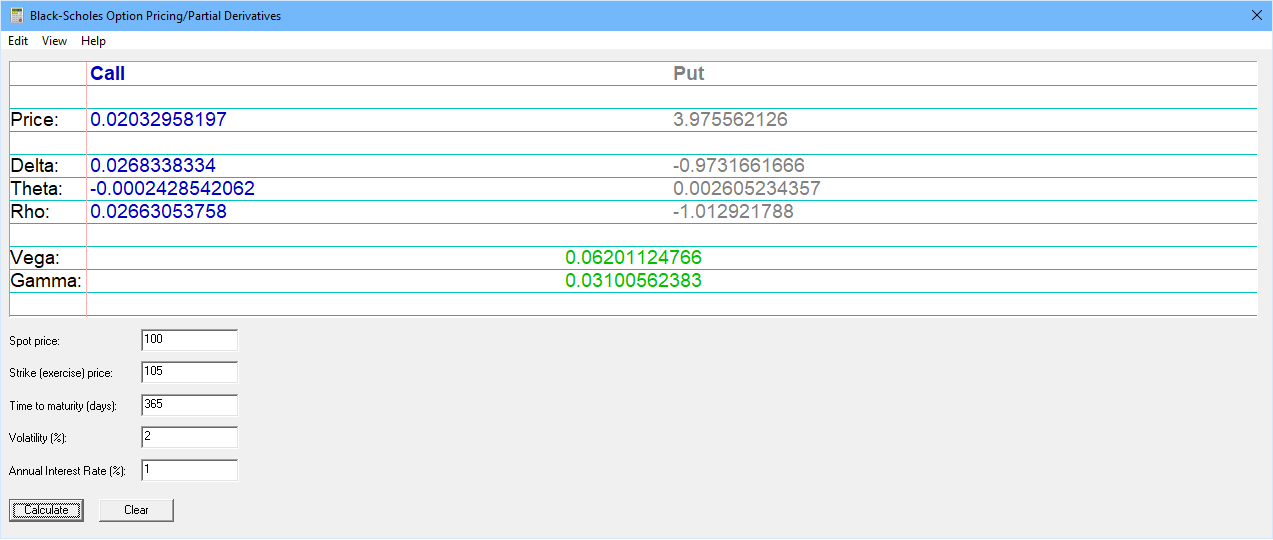### Black-Scholes Option Pricing Calculator

This Black-Scholes Option Pricing Calculator determines the fair market price of European put and call options. It assumes the underlying asset pays no dividends before maturity. We assume no responsibility for the correctness of this software and it should not be used as a basis for trading decisions. It runs on Windows 10 as a desktop application.

The formulas used are:
``` Call option fair price = S0N(d1) - Xe-rtN(d2) Put option fair price = Xe-rtN(-d2) - S0N(-d1) ```

where

``` X is the exercise price S0 is the price of asset at time 0 N(x) is the cumulative normal distribution r is the riskless rate of interest t is the length of time until maturity d1 = ( (ln(S0/X) + rt)/(sigma * t½) ) + (½ * sigma * t½) d2 = d1 - sigma * t½ sigma is the volatility ```

### Screenshot